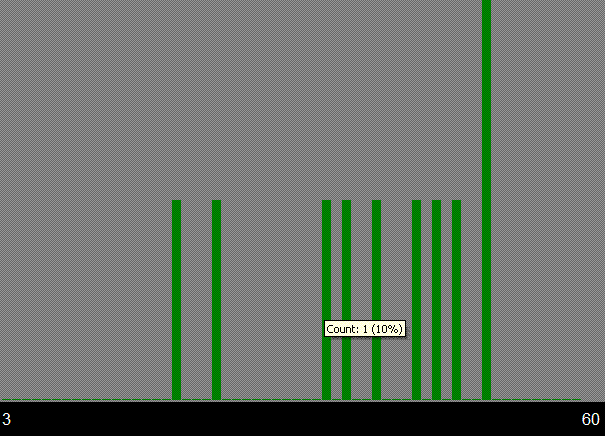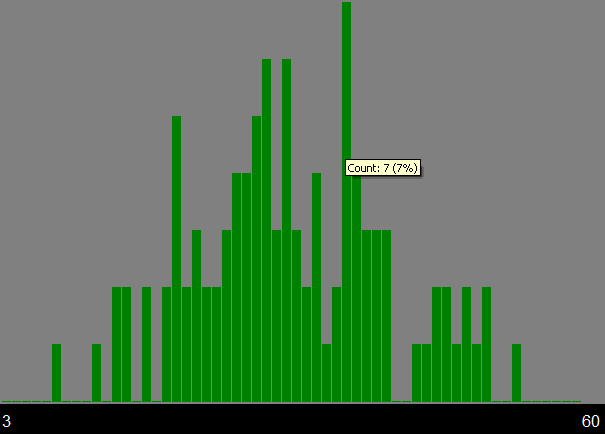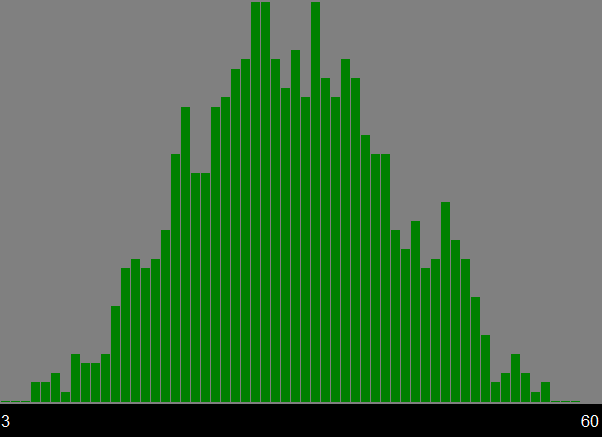Overview When a single die is rolled, any outcome from "1" to "6" is equally likely (assuming a fair die), in other words, the distribution of outcomes is uniform. If two dice are rolled, each pair of outcomes is equally likely, e.g., "1,1" is as likely as "6,6" is as likely as "2,5". However, as craps players know, if we sum the dice, all sums are not equally likely: "7" is the most likely sum, since it arises in 6 out of the 36 outcomes ("1,6", "2,5", "3,4", "4,3", "5,2", and "6,1") and "2" and "12" are the least likely: "2" only arises as a sum for one combination, snake eyes ("1,"1"). What happens as we start rolling not just one die or two dice but five dice or ten dice or five hundred dice and consider the distribution of their sums? Does the same effect happen is instead of six-sided dice we consider dodecahedral (twenty-sided) dice or two-sided "dice" (also known as coins). The Central Limit Theorem tells us that in such cases, the distribution of such sums is normal. Even more interestingly, due to because of the reduction in Coefficient of Variation as more and more dice are summed, the normal distribution gets more and more "squeezed" in the middle as more and more dice are summed. This highlights the advantage that service providers have at increasing scale as addressed in Cloudonomics Laws 3 and 4 implies that service providers that aggregate more demand need fewer resources (the right side of the curve gets compressed towards the mean) and get higher utilization (the left side does the same thing). Basic Flow First, select the number of dice and the number of sides of dice. Second, run any number of random trials, where each trial consists of "rolling" the dice, and including the sum in the histogram plot. You can run 1, 10, 100, or 1000 trials at the push of a button, and keeping the [ENTER] key on your keyboard depressed will repeatedly run that many trials. WARNING: Large numbers of trials with large numbers of dice may take a while, depending on the speed of your computing device. Or, use the "Calculate All" to determine the exact distribution. It only takes 8 six-sided dice to enable well over a million combinations, so random trials are more computationally efficient to assess the distribution that exhaustive combination. Keeping the [ENTER] key depressed enables a hundred thousand or more trials to be conducted fairly rapidly, and also shows the convergence of the distribution to a smooth curve, as shown below in a set of trials involving three twenty-sided dice. After 10 TrialsAfter 100 TrialsAfter 1,000 TrialsAfter 10,000 TrialsAfter 500,000 TrialsAbout This model was written by Joe Weinman in just under 300 lines of code, which are a mixture of HTML, DHTML, ASP.NET 2.0 / Visual Basic, stylesheets, and JavaScript, using Microsoft Visual Web Developer 2008. It has been tested in Firefox 2.0 (Mozilla 5), Safari 3.1, and Internet Explorer 7.0.